In Azerbaijan, the exchange rate follows a fixed exchange rate regime. Moreover, Azerbaijan is a heavily oil-dependent country. That is why in our analysis we would like to use something more flexible and meaningful than the exchange rate. First, we will use the non-oil real effective exchange rate. It represents the non-oil sector and it is more volatile. It is considered one of the fundamentals of the real economy. As for data, we have a set of monthly macroeconomic variables that includes the period from January 2010 to March 2020. We have 123 observations and we have used 100 of them for modelling (training) and 23 of them for prediction errors (testing). As stated above, we want to explain and predict the non-oil real effective exchange rate.

For that we will use some macroeconomic variables in this paper. The first variable of choice is GDP as it is the most important key indicator of the economy. As a proxy suitable for our analysis, we have chosen non-oil real GDP. We have made the variables real by dividing them by the GDP deflator. We expect to find a positive relation with the non-oil real effective exchange rate. The second variable of choice is inflation. However, we have three proxies for inflation in our data set. Our choice needs a bit of elaboration. On hand we have the GDP deflator, monthly CPI change, and annual CPI change. If we look only at the correlation with the non-oil real effective exchange rate, annual CPI performs better than the GDP deflator and monthly CPI respectively with the value -0.79, being highest in absolute terms. The second best is the GDP deflator with the value -0.54. Another way of choosing a variable would be doing simple regressions and selecting the best fit. After three regressions, annual CPI still seems the best choice for modelling. The model with annual CPI has the highest R squared with the value 0.63, the largest coefficient with the lowest p value and the lowest model error. As a result, we choose annual CPI as an inflation variable over other two options.

Next we will try to build a VAR model with the three variables mentioned and test its prediction power for the non-oil real effective exchange rate. We can think of the non-oil real effective exchange rate as a dependent variable and the other two (non-oil real GDP and annual CPI) variables as explanatory variables.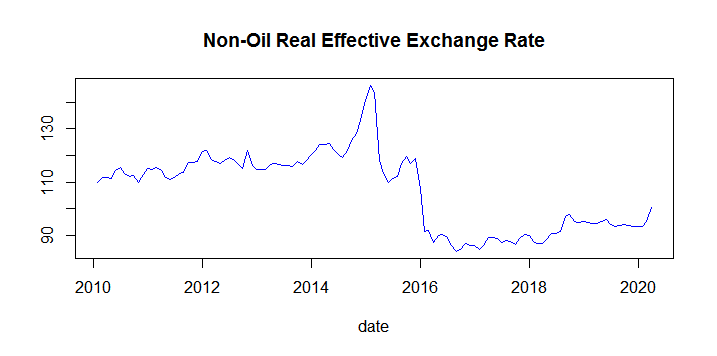Graph 1. Non-oil real effective exchange rate- January 2010 – March 2020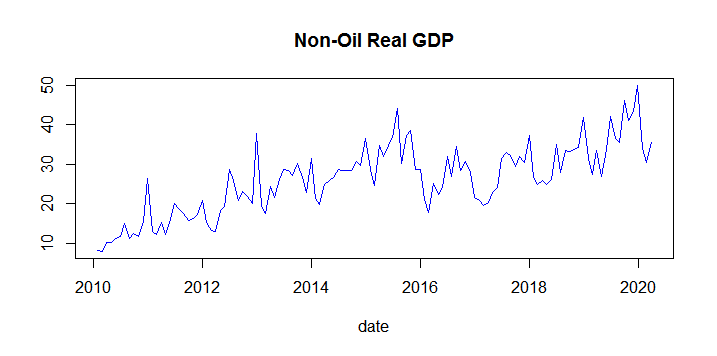Graph 2. Non-oil real GDP- January 2010 – March 2020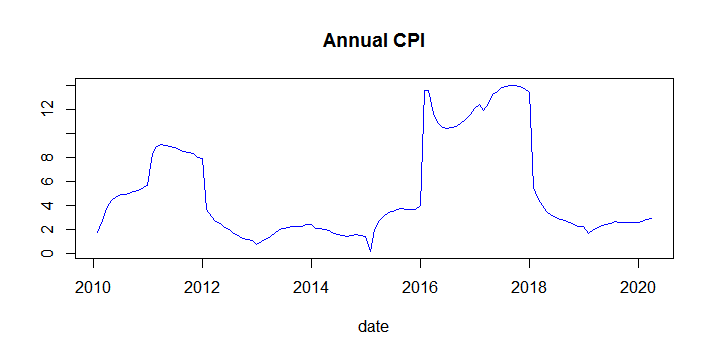Graph 3. Annual CPI- 2010, January-2020, March

To build a VAR model we need to identify how many lags to include. Moreover, it might be useful to conduct Granger causality tests to determine the direction of causality if it exists. To identify the number of lags, first, we use the VARselect function of R and select the lag value automatically.

 AIC HQ SC FPE 6.938899 7.073308 7.272207 1031.815 6.885292 7.120508 7.468581 978.711 6.930743 7.266767 7.764013 1026.087 7.060503 7.497334 8.143754 1172.164 7.114317 7.651955 8.447549 1243.609 7.269297 7.907741 8.85251 1463.571 7.395602 8.134854 9.228796 1678.925 7.518272 8.358331 9.601447 1926.076 7.66557 8.606435 9.998725 2274.587 7.560254 8.601927 10.143391 2097.176

Table 1. Results of VARselect function for each lag

The above table shows the criteria for choosing lags for the VAR model with three variables of interest. The red values are the minimums for each criterion. As shown, two criteria choose one lag and another two criteria choose two for the model. We will try both of them and check how they perform out-of-sample. Out-of-sample prediction errors are calculated as mean squared error.

The model with one lag is used to predict the last 23 members of the non-oil real effective exchange rate till March 2020 and compare to actual values to find mean squared error. Mean squared error from this model is 2.97, more than two times smaller than 7.9, which is the mean squared error from the model with two lags. This shows how lag selection is important to predict data with less error. That is why we will extend our analysis to more lags. We will build 12 models with lag numbers from 1 to 12 and see what happens to mean squared errors when predicting out-of-sample data.

 Mean Squared Error Model 2.97 model 1 7.91 model 2 5.19 model 3 5.92 model 4 2.75 model 5 2.12 model 6 4.43 model 7 3.25 model 8 4.91 model 9 3.3 model 10 16.32 model 11 11.55 model 12

Table 2. Results of predictions for each lag

We would like to explore lag structure more and build more models. However, at some point, the program naturally gives error and the models become meaningless. We could build models with at most 24 lags incorporated. From the table above we see that the model with six lags has lowest out-of-sample prediction error.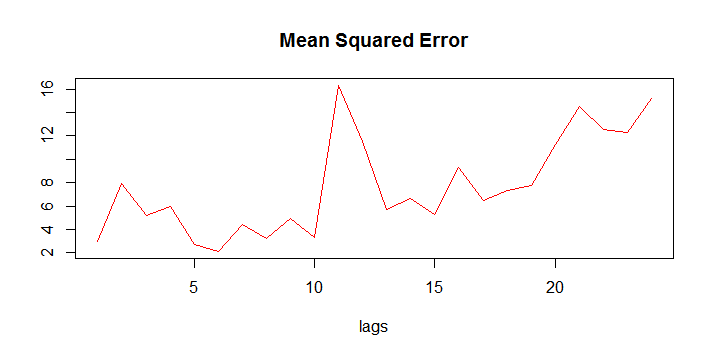Graph 4. Error structure of the models with up to 24 lags

From the graph above, we again confirm that the model with six lags has the best prediction power in our VAR framework with three variables. To compare, random walk performs much worse (with mean squared error 6.77) than the model with six lags. Predictions from random walk are obtained by cumulatively summing up standard normal random variable (standard normal errors).

It is hard to interpret a model with six lags but fortunately we have a method to overcome that. Impulse-response functions are a good way to evaluate the effect of one variable on another.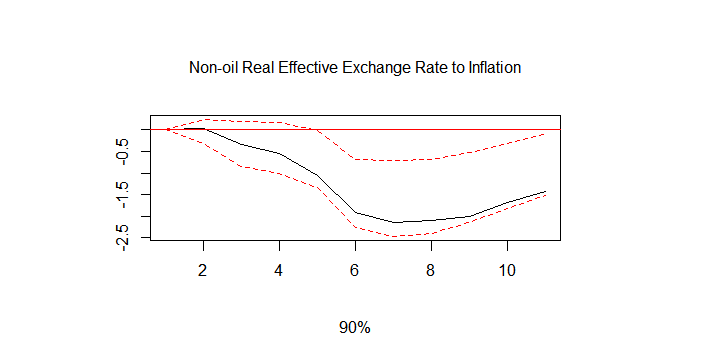Graph 5. Impulse response function (NRER to Inflation)

The graph above means that a positive shock on inflation effects the non-oil effective exchange rate negatively. This is as expected because, as we know, an increase in inflation decreases the value of the currency. Purchasing power compared to other countries drops and because of inflation other countries demand less of a local currency.Graph 6. Impulse response function (NRER to non-oil real GDP)

This graph is not as easy to interpret as inflation. It says the shock on non-oil real GDP first has a positive effect on the non-oil real effective exchange rate which then becomes negative. We expected to have a positive effect. However, for some initial lags, we observe a positive relationship between non-oil GDP and the non-oil real effective exchange rate.

We conducted Granger causality tests and confirmed that the direction of causality is from non-oil real GDP and inflation towards the non-oil real effective exchange rate.  Next we want to add some predictors to our model to see if we can improve the performance. We want to add interest rates to our analysis. However, interest rates are not easy to collect and unreliable in Azerbaijan. Instead, we will use the US federal funds rate as a proxy for the foreign market interest rate. Of course, we expect an effect opposite to that of local interest rates. That is, an increase in the foreign interest rate decreases the demand for local currency and depreciates its value. Once again, first we need to choose the suitable number of lags for our model.Graph 7. Error structure of the models with up to 17 lags

The graph above shows the out-of-sample mean squared error structure per lag. This time the code ran successfully up to 17 lags. It seems that this time model with lower lags perform better. Let’s see the respective numbers.

 Mean Squared Error Model 25.27 model 1 38.09 model 2 3.74 model 3 26.29 model 4 22.51 model 5 22.56 model 6 31.1 model 7 34.12 model 8 61.92 model 9 11.14 model 10 72.49 model 11 37.04 model 12 15.89 model 13 26.66 model 14 30.8 model 15 110.37 model 16 223.92 model 17

Table 3. Results of predictions for each lag

While automatic selection suggests a model with one lag, our approach recommends using a model with three lags. Next we look at the impulse response function.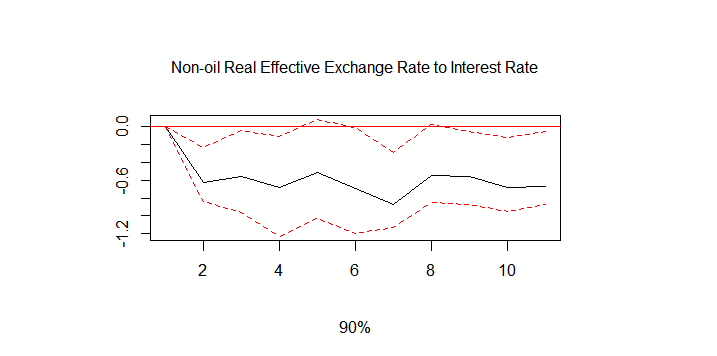Graph 8. Impulse response function (NRER to Foreign Interest Rate)

The graph above shows that a positive shock to the foreign interest rate has a negative effect on the non-oil real effective exchange rate. That fits very well with the theory that an increase in interest rates in the foreign market decreases the demand for the local currency and thus its value depreciates. However, our model with four variables including interest rate and three lags performs a bit worse than the model with three variables and six lags but still better than random walk.

So far we have not mentioned anything about the stationarity of our variables. However, we need to test our variables and check to see if they have a unit root or not. We conducted ADF, PP and KPSS tests to identify whether the variables are stationary or not. To save space, we will not present the test results here. All the tests suggest that our four variables are not stationary and have unit roots. What we do next is to look at the variables in differenced forms. After we differenced the data, conducted tests showed that the variables become stationary. First we will try to build a model with three differenced variables: the non-oil real effective exchange rate (NRER), non-oil real GDP (NRGDP), and inflation(CPI). Then we will add foreign interest rates to look for any more improvement.Graph 9. Error structure of the models with up to 24 lags

The graph above shows the mean squared error structure of of the model with three differenced variables. It seems that the optimal lag number is 8 with the mean squared error 2.8.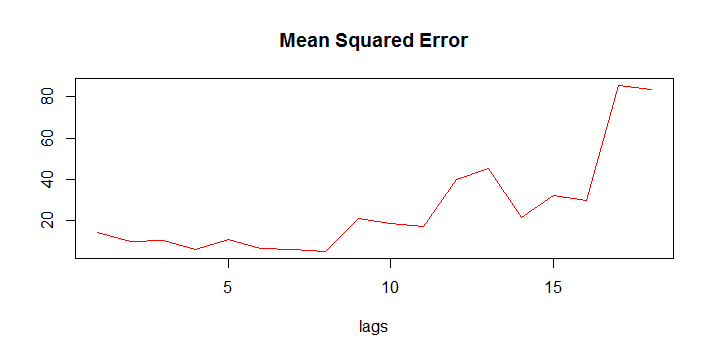Graph 10. Error structure of the models with up to 18 lags

The graph above shows the mean squared error structure of of the model with four differenced variables. It seems that the optimal lag number is again 8 but with the mean squared error 5.13.

In conclusion, the best model with the lowest means squared error is the model with three non-differenced variables and six lags. However, we prefer the second best model with three differenced variables and eight lags since this model uses stationary variables. Adding variables did not necessarily improve our prediction power. We tested some of the other variables but did not see any improvement. We can check more variables in our data set as well to test whether we can improve our mean squared error but we will leave this for further research.